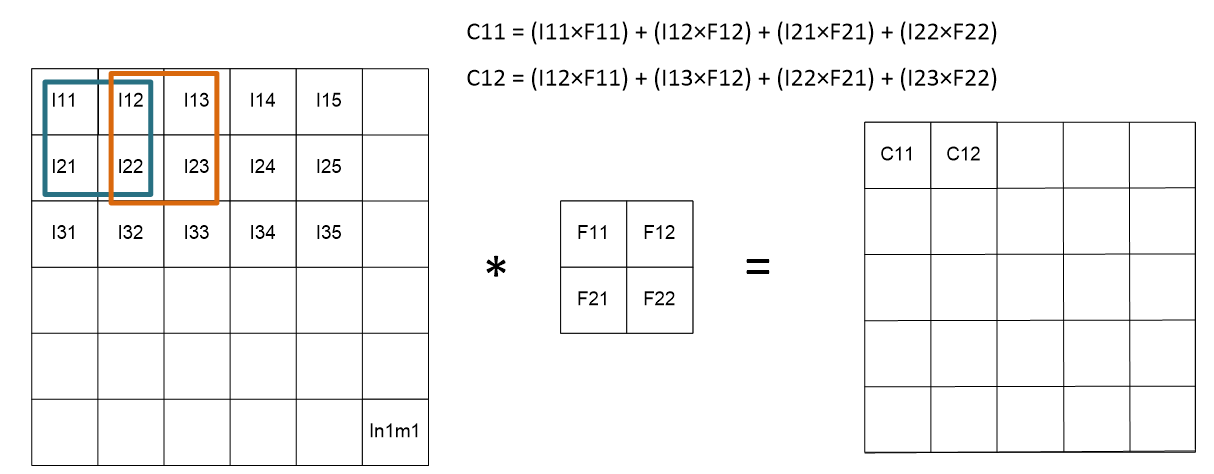Welcome back, readers !! Today we are going to learn about the input layer and convolution layer in deep neural networks.

From today onwards, we will refer to all neural networks, namely CNN, RNN, CRNN, GAN, and other architectures as deep neural networks (DNN), for a few of the upcoming articles.

#### Input layer

It is the first layer of a DNN that allows an image or a signal to enter the architecture for computation. For instance, the resolution of the input image is fixed at 256×256 for further processing.

#### Convolution Layer

The foundation of a DNN is a convolutional layer. It has a number of filters (or kernels), that are used to select and extract the features from images throughout the course of training. Typically, the filters' size is less than the original image. Each filter produces a feature map after it convolves with the image.

For simplicity of understanding, we will assume images as 2D only. So, if the size of an image is n1 × m1 and the size of a filter is n× m2, the output of the convolution layer is (n1 – m1 + 1) × (n2 – m2 + 1).Fig. Working of Convolution Layer

In the above figure, the image's pixel values are represented as I11, I12, I13, ..., In1m1. The pixel values of the filter are represented as F11, F12, F21, and F22. The pixel values of convolved output are represented as C11, C12, and so on, such that,

C11 = (I11×F11) + (I12×F12) + (I21×F21) + (I22×F22)

C11 = (I11×F11) + (I12×F12) + (I21×F21) + (I22×F22)

#### Transposed Convolution Layer

It is just the inverse of what we did with the convolution layer. If the size of an image is n× m1 and the size of a filter is n× m2, the output of the convolution layer is (n+ m1 – 1) × (n+ m2 – 1).

#### Parameters of Convolution Layer

The main parameters of convolution are number of filters, stride and pooling.

• Number of filters
The more the number of filters, the better the efficiency of feature extraction.
• Stride
In layman's terms, the stride is the number of rows or columns the filter jumps to generate the succeeding output pixel value.